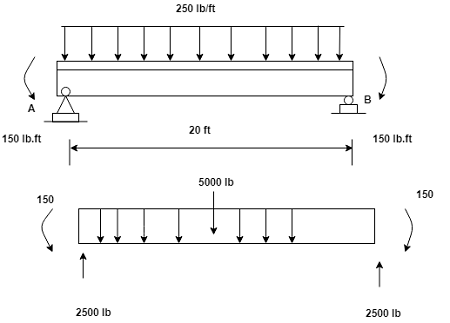# Determine the internal forces (shear, axial and moment) at the midpoint of the loaded beam ( 10...

## Question:

Determine the internal forces (shear, axial and moment) at the midpoint of the loaded beam ( 10 ft from the left end). Include a free-body diagram in your solution. {eq}A_{y} {/eq} = 2500 lb (up). {eq}B_{y} {/eq} = 2500 lb (up)## Moment

The tendency of the force acting on the object to rotate the object about an axis is known as the moment. It is measured in the terms is Newton meter. The moment is the product of the force applied to the object to the perpendicular distance.

Become a Study.com member to unlock this answer! Create your account

Given Data

• Uniform distributed load acting on the complete beam is: {eq}w = 250\;{\rm{lb/ft}} {/eq}.
• Reaction force at point A is: {eq}{A_y} =...Torque in Physics: Equation, Examples & Problems

from

Chapter 3 / Lesson 13
31K

After watching this video, you will be able to explain what torque is and use an equation to calculate torque in simple situations. A short quiz will follow.